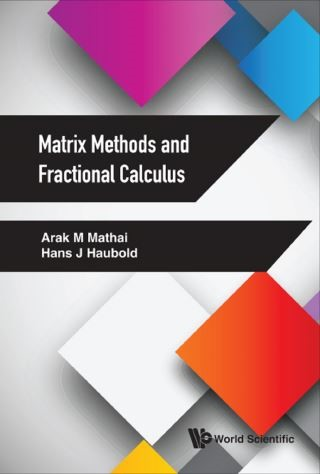## Matrix Methods and Fractional Calculus• Description
• Author
• Info
• Reviews

### Description

Fractional calculus in terms of mathematics and statistics and its applications to problems in natural sciences is NOT yet part of university teaching curricula. This book is one attempt to provide an approach to include topics of fractional calculus into university curricula. Additionally the material is useful for people who do research work in the areas of special functions, fractional calculus, applications of fractional calculus, and mathematical statistics.

Contents:
• Preface
• List of Symbols
• Vector/Matrix Derivatives and Optimization
• Jacobians of Matrix Transformations and Functions of Matrix Argument
• Fractional Calculus and Special Functions
• Fractional Calculus and Fractional Differential Equations
• Kober Fractional Calculus and Matrix-Variate Functions
• Lie Theory and Special Functions
• Selected Topics in Multivariate Analysis

Readership: Graduate students and researchers in all aspects of fractional calculus and its applications.
Keywords:Vector/Matrix Derivatives;Optimization;Jacobians of Matrix Transformations;Multivariate Analysis;Functions of Matrix Argument;Fractional Calculus;Special Functions;Lie Theory;Fractional Differential Equations;Kober Fractional Calculus;Matrix-Variate FunctionsReview:0